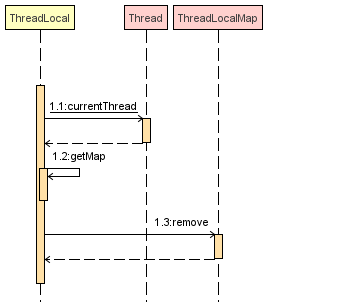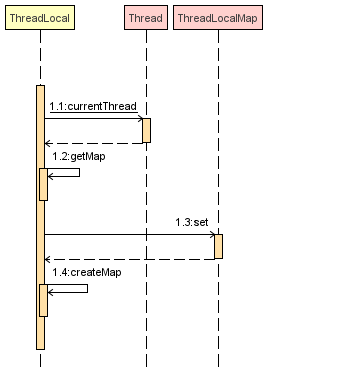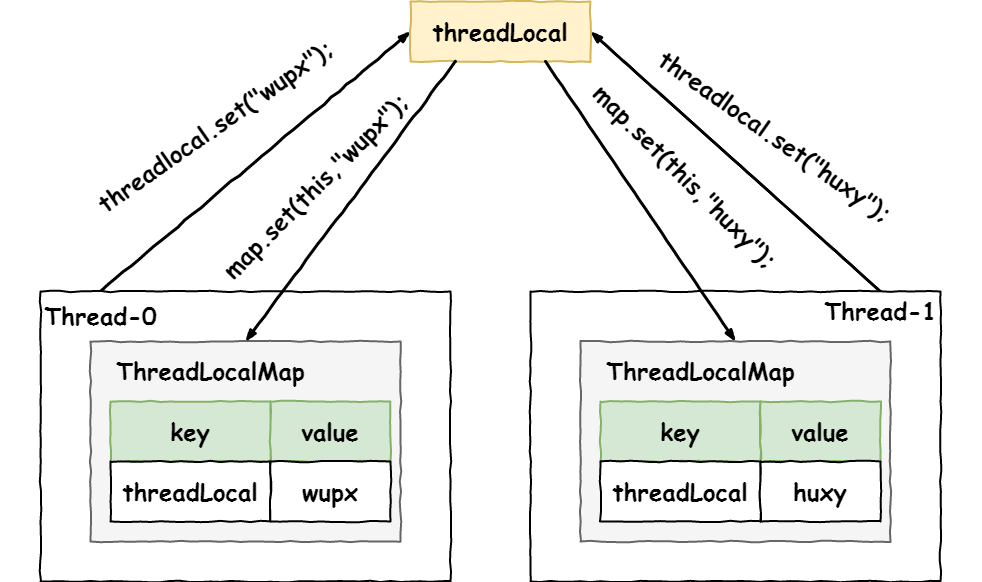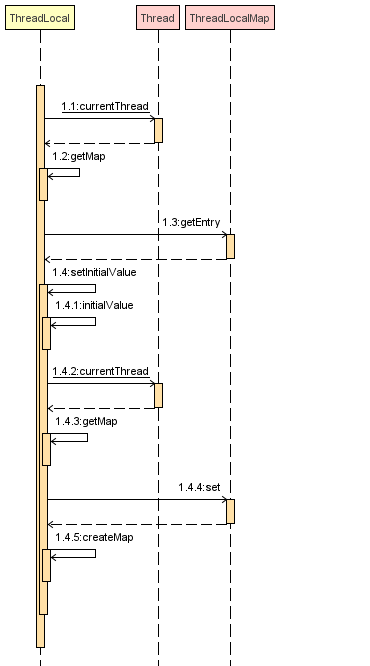``````public class ThreadLocalDemo {
/**
*/

public static void main(String[] args) throws Exception {
}

public void threadLocalTest() throws Exception {
// 主线程设置值

@Override
public void run() {
}
}).start();
// 等待所有线程执行结束
}
}
``````

``````Thread-0线程执行之前，main线程取到的值：wupx

``````

``````// 当前 ThreadLocal 的 hashCode，由 nextHashCode() 计算而来，用于计算当前 ThreadLocal 在 ThreadLocalMap 中的索引位置
private final int threadLocalHashCode = nextHashCode();
// 哈希魔数，主要与斐波那契散列法以及黄金分割有关
private static final int HASH_INCREMENT = 0x61c88647;
// 返回计算出的下一个哈希值，其值为 i * HASH_INCREMENT，其中 i 代表调用次数
private static int nextHashCode() {
}
private static AtomicInteger nextHashCode = new AtomicInteger();
``````

This number represents the golden ratio (sqrt(5)-1) times two to the power of 31 ((sqrt(5)-1) * (2^31)). The result is then a golden number, either 2654435769 or -1640531527.

``````private static final int HASH_INCREMENT = 0x61c88647;

public static void main(String[] args) throws Exception {
int n = 5;
int max = 2 << (n - 1);
for (int i = 0; i < max; i++) {
System.out.print(i * HASH_INCREMENT & (max - 1));
System.out.print(" ");

}
}
``````

``````static class ThreadLocalMap {
/**
* 键值对实体的存储结构
*/
static class Entry extends WeakReference<ThreadLocal<?>> {
// 当前线程关联的 value，这个 value 并没有用弱引用追踪
Object value;

/**
* 构造键值对
*
* @param k k 作 key,作为 key 的 ThreadLocal 会被包装为一个弱引用
* @param v v 作 value
*/
super(k);
value = v;
}
}

// 初始容量，必须为 2 的幂
private static final int INITIAL_CAPACITY = 16;

// 存储 ThreadLocal 的键值对实体数组，长度必须为 2 的幂
private Entry[] table;

private int size = 0;

// 扩容的阈值，默认是数组大小的三分之二
private int threshold;
}
``````

``````/**
*/
public void remove() {
// 返回当前线程持有的 map
if (m != null) {
// 从 map 中清理当前 ThreadLocal 对象关联的键值对
m.remove(this);
}
}
``````

remove 方法的时序图如下所示：remove 方法是先获取到当前线程的 ThreadLocalMap，并且调用了它的 remove 方法，从 map 中清理当前 ThreadLocal 对象关联的键值对，这样 value 就可以被 GC 回收了。

``````/**
* 为当前 ThreadLocal 对象关联 value 值
*
* @param value 要存储在此线程的线程副本的值
*/
public void set(T value) {
// 返回当前线程持有的map
if (map != null) {
map.set(this, value);
} else {
createMap(t, value);
}
}
``````

set 方法的作用是把我们想要存储的 value 给保存进去。set 方法的流程主要是：

• 先获取到当前线程的引用
• 如果 map 不为空，就利用 ThreadLocalMap 的 set 方法将 value 添加到 map 中

set 方法的时序图如下所示：``````/**
*
* @param t 当前线程
*/
}
``````

``````/**
*/
``````

``````/**
* 在 map 中存储键值对<key, value>
*
* @param value 要设置的 value 值
*/
private void set(ThreadLocal<?> key, Object value) {
Entry[] tab = table;
int len = tab.length;
// 计算 key 在数组中的下标
int i = key.threadLocalHashCode & (len - 1);
for (Entry e = tab[i]; e != null; e = tab[i = nextIndex(i, len)]) {

if (k == key) {
e.value = value;
return;
}

// 若 key 是 null，说明 ThreadLocal 被清理了，直接替换掉
if (k == null) {
replaceStaleEntry(key, value, i);
return;
}
}

tab[i] = new Entry(key, value);
int sz = ++size;
// 如果没有元素被清理，那么就要检查当前元素数量是否超过了容量阙值(数组大小的三分之二)，以便决定是否扩容
if (!cleanSomeSlots(i, sz) && sz >= threshold) {
// 扩容的过程也是对所有的 key 重新哈希的过程
rehash();
}
}
````````````/**
*
* @return
*/
public T get() {
if (map != null) {
// 从 map 中拿到 entry
if (e != null) {
@SuppressWarnings("unchecked")
T result = (T) e.value;
return result;
}
}
return setInitialValue();
}
``````

get 方法的主要流程为：

• 先获取到当前线程的引用
• 如果 map 存在，则获取当前 ThreadLocal 对应的 value 值
• 如果 map 不存在或者找不到 value 值，则调用 setInitialValue() 进行初始化

get 方法的时序图如下所示：``````/**
* 返回 key 关联的键值对实体
*
* @return
*/
int i = key.threadLocalHashCode & (table.length - 1);
Entry e = table[i];
// 若 e 不为空，并且 e 的 ThreadLocal 的内存地址和 key 相同，直接返回
if (e != null && e.get() == key) {
return e;
} else {
// 从 i 开始向后遍历找到键值对实体
return getEntryAfterMiss(key, i, e);
}
}
``````

``````/**
* 扩容，重新计算索引，标记垃圾值，方便 GC 回收
*/
private void resize() {
Entry[] oldTab = table;
int oldLen = oldTab.length;
int newLen = oldLen * 2;
// 新建一个数组，按照2倍长度扩容
Entry[] newTab = new Entry[newLen];
int count = 0;

// 将旧数组的值拷贝到新数组上
for (int j = 0; j < oldLen; ++j) {
Entry e = oldTab[j];
if (e != null) {
// 若有垃圾值，则标记清理该元素的引用，以便GC回收
if (k == null) {
e.value = null;
} else {
int h = k.threadLocalHashCode & (newLen - 1);
// 如果发生冲突，使用线性探测往后寻找合适的位置
while (newTab[h] != null) {
h = nextIndex(h, newLen);
}
newTab[h] = e;
count++;
}
}
}
// 设置新的扩容阈值，为数组长度的三分之二
setThreshold(newLen);
size = count;
table = newTab;
}
``````

resize 方法主要是进行扩容，同时会将垃圾值标记方便 GC 回收，扩容后数组大小是原来数组的两倍。

• 方便同一个线程使用某一对象，避免不必要的参数传递
• Spring MVC 的 RequestContextHolder 的实现使用了 ThreadLocal

# 总结

《Java并发编程实战》

https://www.javaspecialists.eu/archive/Issue164.html

https://mp.weixin.qq.com/s/vURwBPgVuv4yGT1PeEHxZQ

Java并发编程学习宝典

Java 并发面试 78 讲